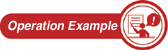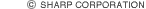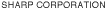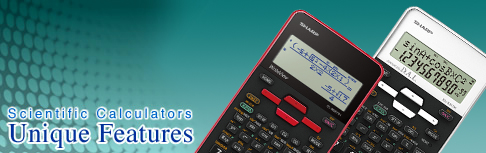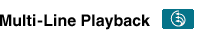SHARP's Multi-Line Playback function lets you recall the steps you have made (up to capacity of memory). This feature is very useful for comparing formulas and recalculating equations.• Comparative Learning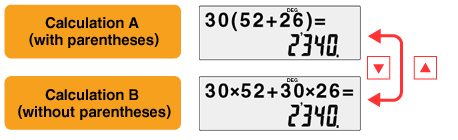The equations are different, but the answers are the same.
 (N.B.) The EL-506T/X/W doesn't display the answer during Multi-Line Playback.
• Edit and Recalculate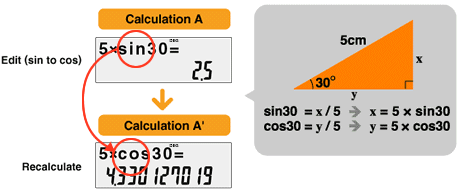Change the previous equation and recalculate.